Summary of the Kolmogorov-Obukhov (1941) theory: overview.

In the last three posts we have summarised various aspects of the Kolmogorov-Obukhov (1941) theory. When considering this theory, the following things need to be borne in mind.

[a] Whether we are working in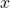-space or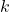-space matters. See my posts of 8 April and 15 April 2021 for a concise general discussion.
[b] In-space the equation of motion (NSE) simply presents us with the problem of an insoluble, nonlinear partial differential equation.
[c] In-space the NSE presents a problem in statistical physics and in itself tells us much about the transfer and dissipation of turbulent kinetic energy.
[d] The Karman-Howarth equation is a local energy balance that holds for any particular value of the distance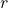between two measuring points.
[e] There is no energy flux between different values of; or, alternatively, through scale.
[f] The energy flux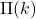is derived from the Lin equation (i.e. in wavenumber space) and cannot be applied in-space.
[g] The maximum value of the energy flux,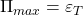(say), is a number, not a function, and can be used (like the dissipation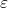) in both-space and-space.
[h] It also matters whether the isotropic turbulence we are considering is stationary or decaying in time.
[g] If the turbulence is decaying in time, then K41B relies on Kolmogorov’s hypothesis of local stationarity. It has been pointed out in a previous post (Part 2 of the present series) that this cannot be the case by virtue of restriction to a range of scales nor in the limit of infinite Reynolds number . See also the supplemental material for .
[h] In-space this is not a problem and the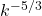spectrum can still be expected , as of course is found in practice.
[i] If the turbulence is stationary, then K41B is exact for a range of wavenumbers for sufficiently large Reynolds numbers. The extent of this inertial range increases with increasing Reynolds numbers.

I have not said anything in this series about the concept of intermittency corrections or anomalous exponents. This topic has been dealt with in various blogs and soon will be again.

 W. D. McComb and R. B. Fairhurst. The dimensionless dissipation rate and the Kolmogorov (1941) hypothesis of local stationarity in freely decaying isotropic turbulence. J. Math. Phys., 59:073103, 2018.
 W. David McComb, Arjun Berera, Matthew Salewski, and Sam R. Yoffe. Taylor’s (1935) dissipation surrogate reinterpreted. Phys. Fluids, 22:61704, 2010.